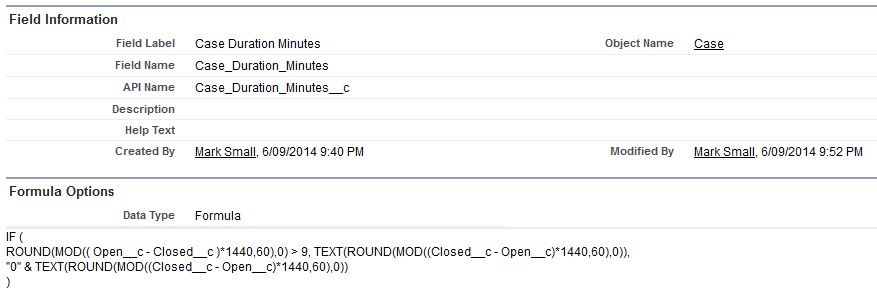# Subtract Two Date Fields to Calculate Duration of Time

If you are faced with the challenge of calculating the duration of time between two dates in Salesforce, this post is for you. Lets say what you want is to take two dates and times, for example 20/01/2014 09:00am and 21/01/2014 10:20am and calculate the duration of time between them in hours and minutes, and display the result like this: 24:20 or 24h:20m.

If we just create a formula field and subtract the two dates, we will end up with something like this:Using a straight subtraction Salesforce gives us the difference of the dates in days and fractions of days. In the example above, it is 44.96, almost 45 days.

To calculate and display the difference in hours and minutes, we have some work to do.

Firstly, lets calculate the number of hours difference between the two dates.
The formula for this calculation is simple, it just takes the difference of the two dates which is the number of days and multiplies by 24 to get the number of hours. It uses the FLOOR function to round down the result to the nearest integer, so if the difference is 44.96 like in the previous example, we want 44 days not 45 days to use in the calculation of the number of hours. We also use an IF statement to add a 0 to the result so we get '09' and not just '9' if the number of hours is less than 10.Then the duration in minutes. This one is a little more complex. We use the MOD function to give us the remainder of a division, in this case the number of minutes that are left over. We also use the ROUND function to remove decimal points. The formula looks a bit more complex as we are firstly testing to see if the number of minutes is less than 10, and if so adding a '0' to the result so we get '07' and not just '7'.Finally, lets put them together. This formula just puts the two previous formula fields together, and also replaces 0:0 (if there was no result) with 00:00.And now in action. The first example is when the open and close dates are on the same days. The result is a calculation of 5 hours and 7 minutes. We have left the calculated fields for the hours and minutes on the page layout, just for demonstration purposes, you would remove them and just leave the final result.The second example is when the open and close dates go over one day. Here the result is 25 hours and 5 minutes.What Certification are you studying for now?

Focus on Force currently provides practice exams and study guides for ten certifications